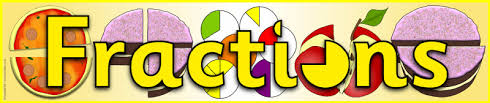3 years ago
jhoelscher
Save
Edit
Host a game
Live GameLive
Homework
Solo Practice
Practice15 QuestionsShow answers
• Question 1
900 seconds
Q. 8/9 - 1/3 =
7/6
5/9
2/3
8/27
• Question 2
900 seconds
Q. What number could be a common denominator for these two fractions?
1/5   and   5/6?
30
11
6
5
• Question 3
900 seconds
Q. What number could be a common denominator for these two fractions?
2/3   and   3/4
3
4
7
12
• Question 4
900 seconds
Q. What number could be a common denominator for these two fractions?
1/5   and   5/6?
30
11
6
5
• Question 5
900 seconds
Q. What must you have to add and subtract fractions?
Common numerators
Different numerators
Different denominators
Common denominators
• Question 6
900 seconds
Q. 2/3 + 1/2 =
6/7
2/6
3/5
7/6
• Question 7
300 seconds
Q. What is the sum of 1/2 + 1/3 + 1/6?
1
3/24
5/6
3/12
• Question 8
900 seconds
Q. Which of these numbers could NOT be a common denominator for these three fractions?
1/3   and 2/5   and   3/10
15
30
60
90
• Question 9
300 seconds
Q. 2/6 + 2/8 =
4/14
4/8
7/12
8/16
• Question 10
300 seconds
Q. 4/8 + 1/3 =
5/11
4/8
5/6
4/11
• Question 11
900 seconds
Q. Aden is helping his dad paint his house. They mixed 5/9 gallons of white paint and 1/6 gallons of blue paint. How many gallons was the mixture in all?
6/18 gallons
13/18 gallons
4/5 gallons
1/6 gallons
• Question 12
900 secondsQ.
1/12
1
2/35
23/25
• Question 13
900 seconds
Q. Solve: 11/12 - ¾
1⅙
1
• Question 14
300 seconds
Q. Subtract and simplify
3/7-2/6
4/42
2/21
1/1
5/13
• Question 15
300 seconds
Q. Subtract and simplify
2/4 - 3/8